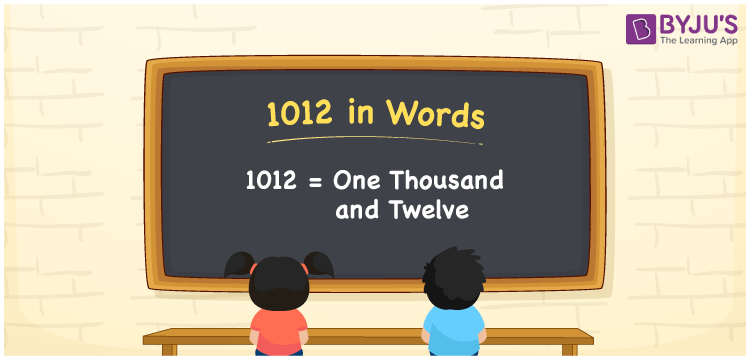1012 in words is One thousand and twelve. For example, if you made a transaction of Rs. 1012, it can be written as “I made a transaction of Rs. One thousand and twelve”. Now, let us discuss how to spell 1012 in English words using the place value system in this article.

 1012 in Words: One Thousand and Twelve. One Thousand and Twelve in Numerical Form: 1012.

## 1012 in English Words## How to Write 1012 in Words?

Below is the place value table for the four-digit number 1012.

 Thousands Hundreds Tens Ones 1 0 1 2

The expanded form of 1012 is as follows:

= 1 × Thousand + 0 × Hundred + 1 × Ten + 2 × One

= 1 × 1000 + 0 × 100 + 1 × 10 + 2 × 1

= 1000 + 10 + 2

= 1012

= One thousand and twelve

Hence, 1012 in words is one thousand and twelve.

1012 in words – One thousand and twelve

Is 1012 an odd number? – No

Is 1012 an even number? – Yes

Is 1012 a perfect square number? – No

Is 1012 a perfect cube number? – No

Is 1012 a prime number? – No

Is 1012 a composite number? – Yes

## Frequently Asked Questions on 1012 in Words

Q1

### How to spell 1012 in English words?

1012 in words is one thousand and twelve.

Q2

### Simplify 1000 + 12, and express it in words.

Simplifying 1000 + 12, we get 1012. Hence, 1012 in words is one thousand and twelve.

Q3

### Is 1012 a prime number?

No, 1012 is not a prime number.